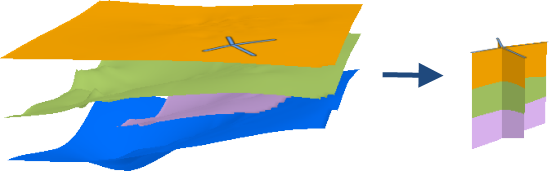# Fence Diagram (3D Analyst)

## Summary

Constructs a vertical cross-section of a collection of surfaces.

## Illustration## Usage

• A fence diagram provides a useful way to visualize the space between multiple overlapping surfaces in 3D space, such as stratigraphic data.

• Bilinear interpolation will be used to derive the height along a raster surface. For TIN surfaces, natural neighbor and linear interpolation options can be specified.

• A table of the x,y,z-values in the fence diagram can be generated with the Stack Profile tool.

## Syntax

`FenceDiagram(in_line_features, in_surface, out_feature_class, {method}, {floor_height}, {ceiling_height}, {sample_distance})`
 Parameter Explanation Data Type in_line_features The line features that will be used to construct the fence diagram. Feature Layer in_surface[in_surface,...] The surfaces that will be used to construct the fence diagram. Mosaic Layer; Raster Layer; TIN Layer out_feature_class The output multipatch that is composed of vertical faces that depict the fence diagram. Feature Class method(Optional) The interpolation method that will be used to obtain z-values from TIN surfaces when constructing the fence diagram. This parameter does not apply to raster surfaces.LINEAR —Linear interpolation will be used. This is the default.NATURAL_NEIGHBORS —Natural neighbors interpolation will be used. String floor_height(Optional) A constant height used to define the lowest height of the fence diagram. Linear Unit ceiling_height(Optional) A constant height used to define the highest height of the fence diagram. Linear Unit sample_distance(Optional) The horizontal distance used for determining the positions where height measurements are interpolated from the underlying surfaces. Linear Unit

## Code sample

FenceDiagram example 1 (Python window)

The following sample demonstrates the use of this tool in the Python window.

``````arcpy.env.workspace = 'c:/data'
arcpy.ddd.FenceDiagram('fence_profile.shp',
['alluvium.tif', 'white_limestone.tif', 'yellow_limestone.tif'],
'fence_diagram.shp', sample_distance='5 Meters')``````

## Licensing information

• Basic: Requires 3D Analyst
• Standard: Requires 3D Analyst